# The Natural Numbers and Induction

Die ganzen Zahlen hat der liebe Gott gemacht; alles andere ist Menschenwerk.

Beloved God made the integers; everything else is the work of human hands.

Leopold Kronecker

Most informal definitions of the natural numbers call them the counting numbers". This is correct, but it's not a mathematical definition. To make a mathematical definition, we need to think a bit more carefully about how counting works.

Activity. Think about how you count. Really think about it. If someone gives you a stack of bills, how you do go about counting it?

### Overview of the Natural Numbers and Induction

• The Natural Numbers (this page).  Natural numbers are the numbers used for counting.
• Proof by Induction. The structure of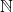provides a handy way to prove statements of the form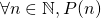.
• Other Uses of Induction.
• Complete Induction. The inductive idea can be pushed into some interesting places.
• The Well-Ordering Principle. Induction, in disguise!
• The Integers and Induction. Technically induction only applies to claims quantified over, but we can usually use it for claims about the integers, too.

### What are the Natural Numbers?

Definition. The natural numbers (denoted) satisfy the following properties:

1. 1 is a natural number.
2. Each natural numberhas a unique successor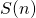, which is a natural number.
3. If two natural numbers have the same successor, they are the same.
4. 1 is nobody's successor.
5. Any natural number can be reached from 1 by a chain of successions.

We call these the Peano axioms, after Giuseppe Peano. They were first published in 1889.

Exercise. Write clauses 2, 3, and 4 as symbolic sentences. Then compute their symbolic and English denials.

We normally represent the natural numbers as dots on a line: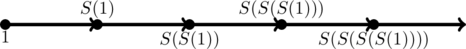where each dot is a natural number, and the arrow points from a number to its successor. We also adopt the symbols 2 for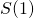, 3 for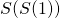, 4 for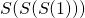, etc.

Each clause of this definition is necessary in order for counting to work. (1) is obvious, but the others are also necessary. Without the others, we might have "number lines" that look very different.

For example, we might have number systems like: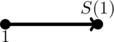which satisfies axioms 1, 3, 4, and 5, but not axiom 2.

Or this:which also satisfies axioms 1, 3, 4, and 5, but not axiom 2.

oror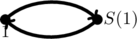Exercise. Identify which Peano axioms each of the "number lines" above satisfies, and which axioms they do not satisfy.

Exercise. Draw a "number line" which satisfies axioms 1, 2, 3, and 4 but not axiom 5.

Peano's definition of the natural numbers is called an axiomatic definition because it's really just a list of axioms, or desirable properties. When we make an axiomatic definition, we specify some of the properties we want the object we're defining has. This is somewhat of a balancing act: if we list too many such properties, it might be that our list is too much to ask for, and no such object exists. If we ask for too few, on the other hand, then maybe there are many different objects which satisfy our definition.

For now, we'll take it on faith that there's a unique system satisfying axioms 1 - 5, which we'll refer to as the natural numbers.